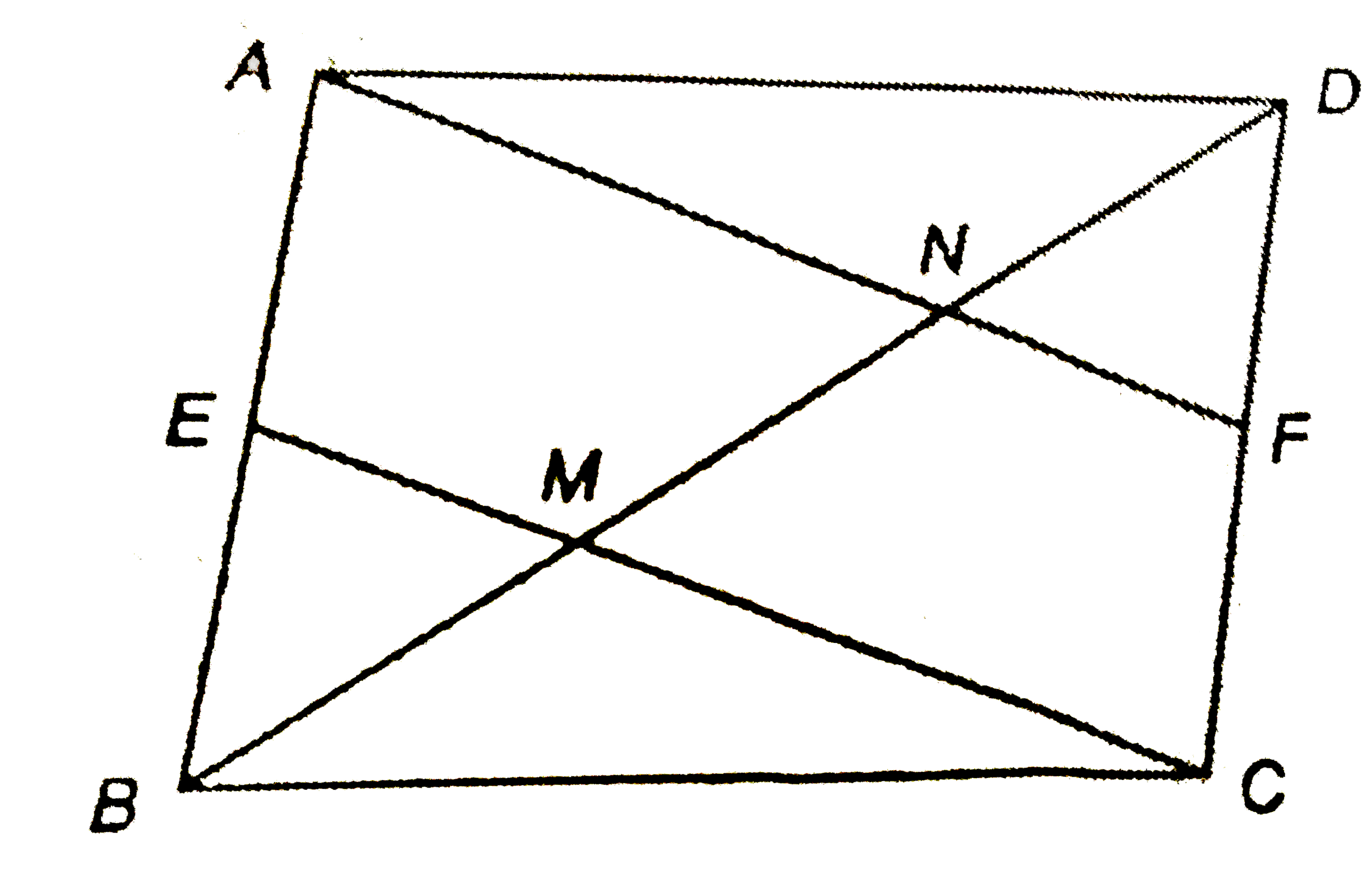# E and F are the mid-points of the sides AB and CD of a parallelogram ABCD. Prove that the line segment AF and CE trisects BD in three equal parts.

43 views

closed
E and F are the mid-points of the sides AB and CD of a parallelogram ABCD. Prove that the line segment AF and CE trisects BD in three equal parts.

by (97.1k points)
selected by

ABCD is a parallelogram
therefore" "AB=DCand AB"||"DC
implies" "2AE=2CFand AE"||"CF
implies" "AE=CFand AE"||"CF
impliessquareAECF is a parallelogram
therefore" "AF"||"ECIn DeltaDCM,
F is the mid-point of DC
and FN"||"CM
therefore N is the mid-point of DM.
implies" "DN=MN" "...(1)
In DeltaBAN,
E is the mid-point of AB.
and" "EM"||"AN
therefore M is the mid-point of BN.
implies" "BM=MN" "...(2)
From eqs, (1) and (2)
" "BM=MN=ND
implies AF and CE, divides the diagonal BD into three equal parts.